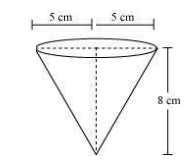# A vessel is in the form of an inverted cone. Its height is 8 cm and the radius of its top, which is open, is 5 cm. It is filled with water up to the brim. When lead shots, each of which is a sphere of radius 0.5 cm are dropped into the vessel, one-fourth of the water flows out. Find the number of lead shots dropped in the vessel.Given

Height of cone (h) = 8 cm

Radius of cone (r) = 5 cm

Radius of sphere (R)= 0.5 cm

Find out

We have to find the number of lead shots dropped in the vessel.

Solution

Let, number of the sphere in cone = n

The number of lead shots = Total Volume of Water overflown/ Volume of Lead shot

The volume of water = Volume of a cone

= 1/3πr2h

= 1/3 × π × 5 × 5 × 8

= 200/3 cm3

The volume of water flows out1/4 (total volume of water)

= (1/4)(200/3)

= 50/3 cm3

Hence, the volume of n spheres = 50/3cm3

Volume of each sphere4/3πr3

= 4/3× π × 0.5 × 0.5 ×0.5

= 6 cm3

Hence, n = Volume of Water overflown/ Volume of Lead shot

n =$$\frac{\frac{50}{3}}{6}$$

n = 100 spheres

The number of lead shots = 100